# KSEEB Solutions for Class 7 Maths Chapter 1 Integers Ex 1.1

Students can Download Chapter 1 Integers Ex 1.1, Question and Answers, Notes Pdf, KSEEB Solutions for Class 7 Maths, Karnataka State Board Solutions help you to revise complete Syllabus and score more marks in your examinations.

## Karnataka State Syllabus Class 7 Maths Chapter 1 Integers Ex 1.1

Question 1.
Following number line shows the temperature in degree Celsius (°C) at different places on a particular day.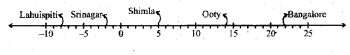a) Observe this number line and write the temperature of the places marked on it.
Solution Places temperature
Solution: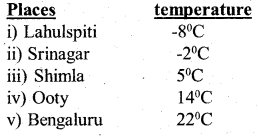b) What is the temperature difference between the hottest and the coldest places among the above ?
Solution:
Hottest temperature among the above places is Bengaluru } = 22°C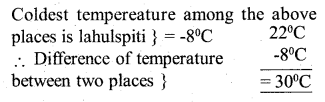c) What is the temperature difference between Lahulspiti and Srinagar ?
Solution: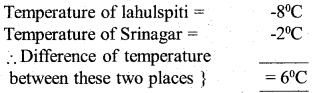d) Can we say the temperature of Srinagar and Shimla taken together is less than the temperature at Shimla? Is it also the temperature at Srinagar?
Solution:
The temperature of Srinagar and Shimla took together = -2°C + 5°C = 3°C
Yes, it is also less than the temperature at Srinagar.Question 2.
In a quiz, positive marks are given for correct answers, and negative marks are given for incorrect answers. If Jack’s scores in five successive rounds were 25, -5, -10, 15, and 10, what was his total at the end?
Solution:
The scores of five successive rounds = 25, -5, -10, 15, 10
Total scores of Jack at the end of the event
= 25 + 15+ 10+ = 50
= -5 -10 = -15
= 35

Question 3.
At Srinagar temperature was -5°C on Monday and then it dropped by 2°C on Tuesday. What was the temperature of Srinagar on Tuesday? On Wednesday, it rose by 4°C. What was the temperature on this day?
Solution:
The temperature on Monday at Srinagar = -5°C
The temperature on Tuesday at Srinagar = -5 – 2 = -7° C
The temperature on Wednesday at Srinagar = -7 + 4 = -3° C

Question 4.
A plane is flying at a height of 5000 m above the sea level. At a particular point, it is exactly above a submarine floating 1200m below the sea level. What is the vertical distance between them?
Solution:Height of the plane flying above sea level = + 5000m
Level of the submarine below sea = – 1200m
Vertical distance between them = 5000m – (- 1200m) = 5000 + 1200 = 6200m.Question 5.
Mohan deposits 2,000 in his bank account and withdraws 1,642 from it, the next day. If the withdrawal of amount from the account is represented by a negative integer, then how will you represent the amount deposited? Find the balance in Mohan’s account after the withdrawal.
Solution: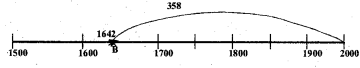From the above number line
Distance from A to B = 20 kms
Distance from B to C = 30 kms
Final position is = C
Distance from A to C = – 10 kms
From the number line, the Balance amount represents the distance B to A.
B to A = Rs. 358.

Question 6.
Rita goes 20 km towards east from a point A to the point B. From B, she moves 30 km towards west along the same road. If the distance towards east is represented by a positive integer then, how will you represent the distance travelled towards west? By which integer will you represent her final position from A?
Solution: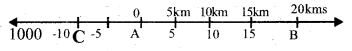From the above number line
Distance from A to B = 20 kms
Distance from B to C = 30 kms
Final position is = C
Distance from A to C = -10 kms
From the original starting point.

Question 7.
In a magic square each row, column and diagonal have the same sum. Check which of the following is a magic square.
Solution:From the (i) square
1st row 5 + (-1) + (-4) = 0
2nd row -5 + -2 + 7 = 0
3rd row 0 + 3 + -3 = 0
1st column 5 + -5 + 0 = 0
2nd column -1 + -2 + 3 = 0
3rd column -4 + 7 – 3 = 0
1st Diagonal 5 + -2 + -3 = 0
2nd Diagonal 0 + -2 + -4 = -6
∴ It is not a magic square.(ii) 1st row 1 + -10 + 0 = -9
2nd row – 4 + -3 – 2 = -9
3rd row -6 + 4 – 7 = -9
1st column 1 + – 4 – 6 = -9
2nd column -10 + -3 + 4 = -9
3rd column 0 + – 2 – 7 = -9
1st Diagonal – 6 – 3 + 0 = -9
2nd Diagonal 1 – 3 – 7 = -9
∴ It is a magic square.

Question 8.
Verify a – (-b) = a + b for the following values of a and b.
i) a = 21, b = 18ii) a = 118, b = 125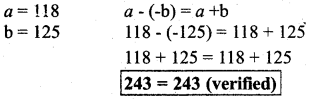iii) a = 75, b = 84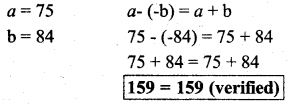iv) a = 28, b = 11Question 9.
Use the sign of >, < or = in the box to make the statements true.
Solution:Question 10.
A water tank has stepped inside it. A monkey is sitting on the topmost step (i.e., the first step). The water level is at the ninth step.
Solution:
i) He jumps 3 steps down and then jumps back 2 steps up. In how many jumps will he reach the water level?
Solution:
Monkey jumps down = 3 steps
Monkey again jumps up = 2 steps
presenting this on number line,
Initial position = 1st step
Final position = 9th step
Representing this on number line,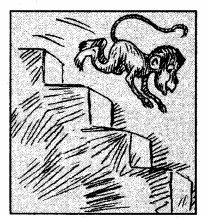First Jump = 1 + 3 = 4 steps
Second Jumb = 4 – 2 = 2 steps
Third Jump = 2 + 3 = 5 steps
Fourth Jump = 5 – 2 = 3 steps
Fifth Jump = 3 + 3 = 6 steps
Sixth Jump = 6 – 2 = 4 steps
Seventh Jump = 4 + 3 = 7 steps
Eight Jump = 7 – 2 = 5 steps
nineth Jump = 5 + 3 = 8 steps
tenth Jump = 8 – 2 = 6 steps
Eleventh Jump = 6 + 3 = 9 steps
∴ Monkey took 11 Jumps to reach 9 steps.ii) After drinking water, he wants to go back. For this, he jumps 4 steps up and then jumps back 2 steps down in every move. In how many jumps will he reach back the top step?
Solution:
Initial position = 9th step. Final position = 1st step
Monkey Jumps up = 4 steps
Monkey Jumps down = 2 stepsFirst Jump = 9 – 4 = 5th step
Second Jumb = 5 + 2 = 7th step
Third Jump = 7 – 4 = 3th step
Fourth Jump = 3 + 2 = 5th step
Fifth Jump = 5 – 4 = 1st step
∴ Monkey took 5 Jumps to reach back

iii) If the number of steps moved down is represented by negative integers and the number of steps moved up by positive integers, represent his moves in part (i) and (ii) by completing the following; In (a) the sum (-8) represents going down by eight steps. So, what will the sum 8 in (b) represent?
(a) -3 + 2 – ……. = -8
-3 + 2 – 3 + 2 – 3 + 2 – 3 + 2 – 3 + 2 – 3 + 2 – 3 + 2 – 3 + 2 = -8

(b) 4 – 2 + …. = 8
Thus, Sum 8 in (b) represents going up by eight steps.

error: Content is protected !!